# A Blend Example[Page 132]

A petroleum company produces three grades of motor oilsuper, premium, and extrafrom three components. The company wants to determine the optimal mix of the three components in each grade of motor oil that will maximize profit. The maximum quantities available of each component and their cost per barrel are as follows :

Component

Maximum Barrels Available/Day

Cost/Barrel

1

4,500

\$12

2

2,700

10

3

3,500

14

To ensure the appropriate blend, each grade has certain general specifications. Each grade must have a minimum amount of component 1 plus a combination of other components, as follows:

Grade

Component Specifications

Selling Price/Barrel

Super

At least 50% of 1

\$23

Not more than 30% of 2

Premium

At least 40% of 1

20

Not more than 25% of 3

Extra

At least 60% of 1

18

At least 10% of 2

The company wants to produce at least 3,000 barrels of each grade of motor oil.

#### Decision Variables

The decision variables for this problem must specify the quantity of each of the three components used in each grade of motor oil. This requires nine decision variables, as follows: x ij = barrels of component i used in motor oil grade j per day, where i = 1, 2, 3 and j = s (super), p (premium), e (extra). For example, the amount of component 1 in super motor oil is x 1 s . The total amount of each grade of motor oil will be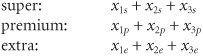#### The Objective Function

The company's objective is to maximize profit. This requires that the cost of each barrel be subtracted from the revenue obtained from each barrel. Revenue is determined by multiplying the selling price of each grade of motor oil by the total barrels of each grade produced. Cost is achieved by multiplying the cost of each component by the total barrels of each component used: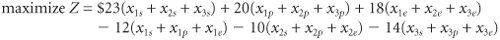"Profit" is maximized in the objective function by subtracting cost from revenue .

[Page 133]

Combining terms results in the following objective function:

maximize Z = 11 x 1 s + 13 x 2 s + 9 x 3 s + 8 x 1 p + 10 x 2 p + 6 x 3 p + 6 x 1 e + 8 x 2 e + 4 x 3 e

#### Model Constraints

This problem has several sets of constraints. The first set reflects the limited amount of each component available on a daily basis:

x 1 s + x 1 p + x 1 e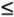4,500 bbl.

x 2 s + x 2 p + x 2 e2,700 bbl.

x 3 s + x 3 p + x 3 e3,500 bbl.

The next group of constraints is for the blend specifications for each grade of motor oil. The first specification is that super contain at least 50% of component 1, which is expressed as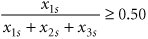This constraint says that the ratio of component 1 in super to the total amount of super produced, x 1 s + x 2 s + x 3 s , must be at least 50%. Rewriting this constraint in a form more consistent with linear programming solution results in

Standard form requires that fractional relationships between variables be eliminated .

x 1 s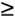0.50( x 1 s + x 2 s + x 3 s )

and

0.50 x 1 s 0.50 x 2 s 0.50 x 3 sThis is the general form a linear programming constraint must be in before you can enter it for computer solution. All variables are on the left-hand side of the inequality, and only numeric values are on the right-hand side.

The constraint for the other blend specification for super grade, not more than 30% of component 2, is developed in the same way: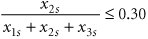and

0.70 x 2 s - 0.30 x 1 s - 0.30 x 3 sThe two blend specifications for premium motor oil are

60 x 1 p - 0.40 x 2 p - 0.40 x 3 p0.75 x 3 p - 0.25 x 1 p - 0.25 x 2 pThe two blend specifications for extra motor oil are

0.40 x 1 e - 0.60 x 2 e - 0.60 x 3 e0.90 x 2 e - 0.10 x 1 e - 0.10 x 3 eThe final set of constraints reflects the requirement that at least 3,000 barrels of each grade be produced:

x 1 s + x 2 s + x 3 s3,000 bbl.

x 1 p + x 2 p + x 3 p3,000 bbl.

x 1 e + x 2 e + x 3 e3,000 bbl.

[Page 134]

#### Model Summary

The complete linear programming model for this problem is summarized as follows: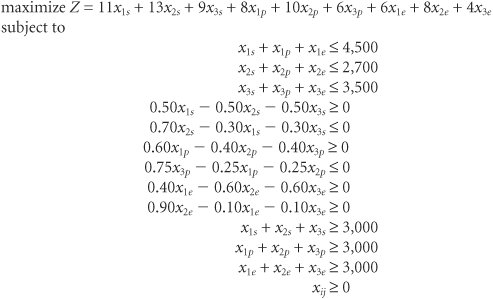#### Computer Solution with Excel

The Excel spreadsheet solution for this blend example is shown in Exhibit 4.17. Solver is shown in Exhibit 4.18. The decision variables are located in cells B7:B15 in Exhibit 4.17. The total profit is computed in cell C16, using the formula = SUMPRODUCT(B7:B15,C7:C15 ), which is also shown on the formula bar at the top of the spreadsheet. The constraint formulas are embedded in cells H6 through H17. Notice that we did not develop an array of constraint coefficients on the spreadsheet for this model; instead, we typed the constraint formulas directly into cells H6:H17, which seemed easier. For example, the constraint formula in cell H6 is = B7+B10+B13 , and the constraint formula in cell H9 is = .5*B7 .5*B8 .5*B9 . The remaining cells in column H have similar constraint formulas.

##### Exhibit 4.17.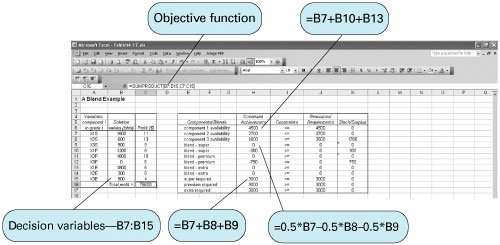[Page 135]
##### Exhibit 4.18.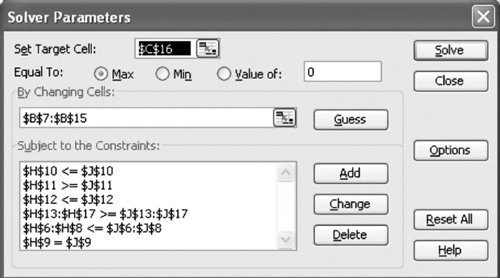#### Solution Analysis

The solution is

x 1 s = 1,500 bbl.

x 2 s = 600 bbl.

x 3 s = 900 bbl.

x 1 p = 1,200 bbl.

x 2 p = 1,800 bbl.

x 1 e = 1,800 bbl.

x 2 e = 300 bbl.

x 3 e = 900 bbl.

Z = \$76,800

Summarizing these results, 3,000 barrels of super grade, premium, and extra are produced. Also, 4,500 barrels of component 1, and 2,700 barrels of component 2, and 1,800 barrels of component 3 are used. (This problem also contains multiple optimal solutions.)

Exhibit 4.19 shows the sensitivity report for our Excel solution of the blend problem. Notice that the shadow price for component 1 is \$20. This indicates that component 1 is by far the most critical resource for increasing profit. For every additional barrel of component 1 that can be acquired , profit will increase by \$20. For example, if the current available amount of component 1 were increased from 4,500 barrels to 4,501 barrels, profit would increase from \$76,800 to \$76,820. (This dual price is valid up to 6,200 barrels of component 1, the upper limit for the sensitivity range for this constraint quantity value.)

##### (This item is displayed on page 136 in the print version)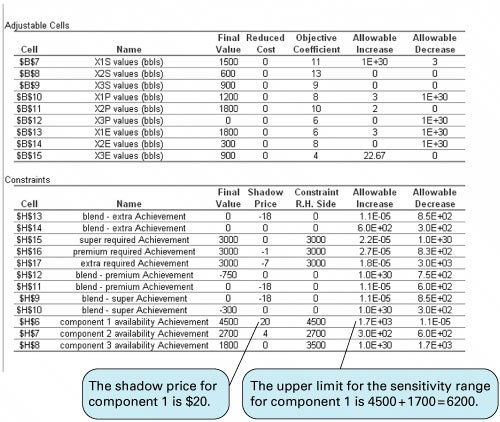Recall that the shadow price is the marginal economic value of one additional unit of a resource .

In the refinery industry, different grade stocks of oil and gasoline are available based on the makeup and quality of the crude oil that is received. Thus, as crude oil properties change, it is necessary to change blend requirements. Component availability changes as well. The general structure of this model can be used on a daily basis to plan production based on component availability and blend specification changes.Introduction to Management Science (10th Edition)
ISBN: 0136064361
EAN: 2147483647
Year: 2006
Pages: 358

Similar book on Amazon

flylib.com © 2008-2017.
If you may any questions please contact us: flylib@qtcs.net